# Penetrable sphere model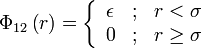$\Phi_{12}\left( r \right) = \left\{ \begin{array}{ccc} \epsilon & ; & r < \sigma \\ 0 & ; & r \ge \sigma \end{array} \right.$
where$\Phi_{12}(r)$ is the intermolecular pair potential,$\epsilon$ is the hight of the repulsive core,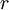$r$ is the distance between site 1 and site 2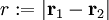$r := |\mathbf{r}_1 - \mathbf{r}_2|$ and$\sigma$ is the radius of the core. Note: an earlier, different, penetrable sphere model was proposed, but is now known as the Widom-Rowlinson model. This model can be seen as the limiting case of the Gaussian overlap model where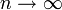$n \rightarrow \infty$. In the limit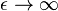$\epsilon \rightarrow \infty$ one has the hard sphere model.